• 一、分支定界法相关概念、 二、分支定界法求解整数规划步骤、 三、分支定界理论分析、 四、分支过程示例
文章目录一、分支定界法相关概念二、分支定界法求解整数规划步骤三、分支定界理论分析四、分支过程示例

一、分支定界法相关概念

分支定界法相关概念 :
分支 : 将一个问题不断细分为 若干子问题 , 之后逐个讨论子问题 ;
定界 : 分支很多的情况下 , 需要讨论的情况也随之增多 , 这里就需要定界 , 决定在什么时候不在进行分支 ; 满足 ① 得到最优解 , ② 根据现有条件可以排除最优解在该分支中 , 二者其一 , 就可以进行定界 ;
定界的作用是 剪掉没有讨论意义的分支 , 只讨论有意义的分支 ;

二、分支定界法求解整数规划步骤

分支定界法求解整数规划步骤 :
( 1 ) 求 整数规划 的 松弛问题 最优解 :
如果 该 最优解就 是整数 , 那么得到整数规划最优解 ;
如果 该 最优解 不是整数 , 那么转到下一个步骤 分支 与 定界 ;

( 2 ) 分支 与 定界 :
任选一个 非整数解变量 $x_i$ , 在 松弛问题 中加上约束 :
$x_i \leq [x_i]$ 和 $x_i \geq [x_i] + 1$
形成 两个新的 松弛问题 , 就是两个分支 ;
上述分支 , 分的越细致 , 限制条件越多 , 同时 最优解的质量就越差 ;
新的分支松弛问题特征 :

原问题求 最大值 时 , 目标值 是 分支问题 的上界 ;

原问题求 最小值 时 , 目标值 是 分支问题 的下界 ;

分支 $1$ 的最优解是 $x^*$ , 将最优解代入目标函数后得到最优值 $f_1$ ;
分支 $2$ 的最优解是 $y^*$ , 将最优解代入目标函数后得到最优值 $f_2$ ;
如果目标函数求最大值 , 那么就讨论 $f_1, f_2$ 谁更大 ;

检查 分支松弛问题 的 解 及 目标函数值 :
① 得到最优整数解 : 如果该分支的 解 是 整数 , 并且 目标函数值 大于等于 其它分支的目标值 , 则剪去其它分支 , 停止计算 ;
② 没有得到最优整数解 : 如果该分支的 解 是 小数 , 并且 目标函数值 大于 整数解的目标值 , 需要 继续进行分支 , 直到得到最优解 ;

三、分支定界理论分析

假设考虑 分支 $1$ 松弛问题 , 每次都要给问题找到一个界 , 开始先使用观察法找到一个界 , 找到一个整数解 $f$ , 将该解代入目标函数 , 然后在 不断地计算中, 修改该界 ;
假设 分支 $1$ 松弛问题 目标函数 求最大值 ,
如果求解 分支 $1$ 松弛问题 最优解 恰好也是一个整数解 $f_0$ ,  如果 $f_0 > f$ , 此时需要重新定界 , 将 $f_0$ 作为新的界来讨论 ;
根据新的界来看 分支 $2$ 松弛问题是否需要讨论 , 求出 分支 $2$ 的最优解  对应的 目标函数最优值 $f^*$ ;
如果 分支 $2$ 的最优值 小于 分支 $1$ 的最优值 $f^* \leq f^0$ , 此时分支 $2$ 不用再进行分支了 , 再进行分支 , 其最优值会越来越差 ;
定界法的作用是将不符合条件的分支剪去 ;

四、分支过程示例

如上篇博客 【运筹学】整数规划 ( 整数规划问题解的特征 | 整数规划问题 与 松弛问题 示例 ) 中 , 求解如下 整数规划 解 :
$\begin{array}{lcl} \rm maxZ = x_1 + x_2 \\\\ \rm s.t\begin{cases} \rm 14 x_1 + 9x_2 \leq 51 \\\\ \rm -6 x_1 + 3x_2 \leq 1 \\\\ \rm x_1, x_2 \geq 0 \ 并且为整数 \end{cases}\end{array}$

其对应的松弛问题是 : 去掉整数解限制 ;
$\begin{array}{lcl} \rm maxZ = x_1 + x_2 \\\\ \rm s.t\begin{cases} \rm 14 x_1 + 9x_2 \leq 51 \\\\ \rm -6 x_1 + 3x_2 \leq 1 \\\\ \rm x_1, x_2 \geq 0 \end{cases}\end{array}$

分支都是基于松弛问题进行的 , 为松弛问题分支 , 组成两个新的松弛问题 ;

下图是求解结果 ( 图解法 ) :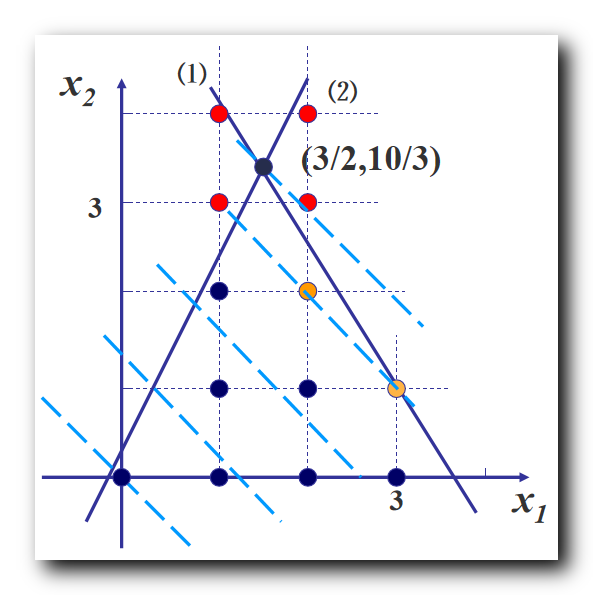最优解 $x_1 = \cfrac{3}{2}$ , 分别为 $x_1$ 添加 $x_i \leq [x_i]$ 和 $x_i \geq [x_i] + 1$  约束 , 形成两个新的线性规划 ;

分支 $1$ 整数规划 : 添加 $x_i \leq [x_i]$ 约束 , 即 $x_1 \leq 1$ ;
$\begin{array}{lcl} \rm maxZ = x_1 + x_2 \\\\ \rm s.t\begin{cases} \rm 14 x_1 + 9x_2 \leq 51 \\\\ \rm -6 x_1 + 3x_2 \leq 1 \\\\ \rm x_1 \leq 1 \\\\ \rm x_1, x_2 \geq 0 \end{cases}\end{array}$

分支 $2$ 整数规划 : 添加 $x_i \geq [x_i] +1$ 约束 , 即 $x_1 \geq 2$ ;
$\begin{array}{lcl} \rm maxZ = x_1 + x_2 \\\\ \rm s.t\begin{cases} \rm 14 x_1 + 9x_2 \leq 51 \\\\ \rm -6 x_1 + 3x_2 \leq 1 \\\\ \rm x_1 \geq 2 \\\\ \rm x_1, x_2 \geq 0 \end{cases}\end{array}$

这样就将一个线性规划 , 分解成了两个问题分支 , 分别找两个分支问题的所有整数解 ;
整数规划的整数解 , 肯定在上述两个分支之一中 , 中间将一部分可行域排除在外了 , 就是下图中两个红色箭头之间的可行域部分 , 被排除掉的部分肯定没有整数解 , 都是小数 ;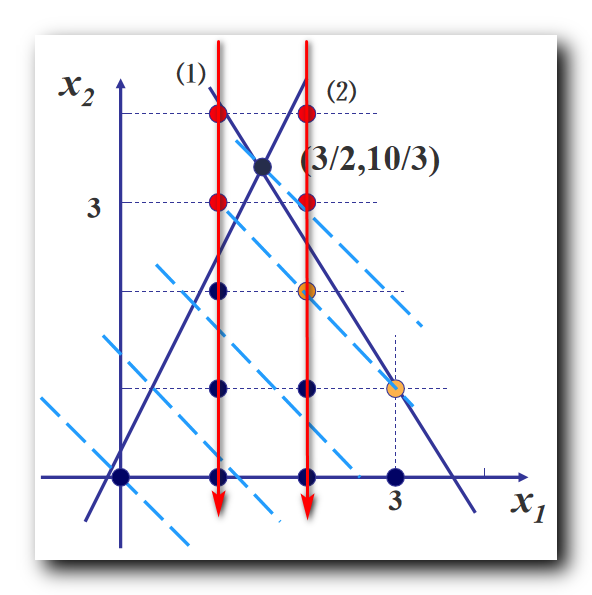展开全文整数规划问题
• 六、分支定界法、 1、整数规划概念、 2、分支定界法求解整数规划步骤、 3、分支定界理论分析、 七、分支过程示例、 八、分支定界法求整数规划示例、 1、分支定界法求整数规划示例、 2、求整数规划的松弛问题及最优解...
文章目录一、整数规划1、整数规划概念2、整数规划分类二、整数规划示例三、整数规划解决的核心问题四、整数规划问题解的特征五、整数规划问题 与 松弛问题 示例六、分支定界法1、整数规划概念2、分支定界法求解整数规划步骤3、分支定界理论分析七、分支过程示例八、分支定界法求整数规划示例1、分支定界法求整数规划示例2、求整数规划的松弛问题及最优解3、第一次分支操作4、第二次分支操作5、第三次分支操作6、整数规划最优解

一、整数规划

1、整数规划概念

线性规划 使用 单纯形法求解 , 线性规划中的 运输规划 使用 表上作业法 求解 ;
之前讨论的都是线性规划问题 , 非线性规划如何求解 , 没有给出具体的方法 ;

整数规划问题 : 要求 一部分 或 全部 决策变量 取值整数 的规划问题 , 称为整数规划 ;
整数规划问题的松弛问题 : 不考虑 整数变量条件 , 剩余的 目标函数 和 约束条件 构成的线性规划问题 称为 整数规划问题的松弛问题 ;
整数线性规划 : 如果上述 整数规划问题的松弛问题 是线性规划  , 则称该整数规划为 整数线性规划 ;

整数规划与之前的线性规划多了一个约束条件 , 变量大于等于 $0$ , 并且都是整数 ;

整数线性规划数学模型一般形式 :
$\begin{array}{lcl} \rm maxZ = \sum_{j = 0}^{n} c_j x_j \\\\ \rm s.t\begin{cases} \rm \sum_{j = 1}^{n} a_{ij} x_j = b_i \ \ \ ( \ i = 1, 2, \cdots , m \ ) \\\\ \rm x_j \geq 0 \ \ \ ( \ j = 1, 2, \cdots , n \ ) 部分 或 全部 为整数 \end{cases}\end{array}$

2、整数规划分类

整数线性规划分为以下几类 : ① 纯整数线性规划 , ② 混合整数线性规划 , ③ 0-1 型整数线性规划 ;

① 纯整数线性规划 : 全部决策变量都 必须取值整数 的 整数线性规划 ;
② 混合整数线性规划 : 决策变量中有一部分 必须 取整数值 , 另一部分 可以不 取值整数值 的 整数线性规划 ;
③ 0-1 型整数线性规划 : 决策变量 只能取值 $0$ 或 $1$ 的整数线性规划 ;

二、整数规划示例

资金总额 $\rm B$ , 有 $n$ 个投资项目 , 项目 $j$ 所需的投资金额 是 $a_j$ , 预期收益是 $c_j$ , $j = 1,2,\cdots,n$ ;
投资还有以下附加条件 :
① 如果投资项目 $1$ , 必须投资项目 $2$ ; 反之如果投资项目 $2$ , 没有限制 ;
② 项目 $3$ 和 项目 $4$ 必须至少选 $1$ 个 ;
③ 项目 $5,6,7$ 只能选择 $2$ 个 ;

决策变量分析 : 选择合适的 决策变量 与 决策变量取值 ;
选取变量 , 使得变量的一组取值 , 能更好对应线性规划问题的解决方案 ;
每个项目有对应的两个选择 , 投资 / 不投资 , 分别使用 $1$ 和 $0$ 表示 ;
令 $x_j$ 表示第 $j$ 个项目的投资选择 , 投资 $1$ , 不投资 $0$ ; ( $j = 1,2, \cdots, n$ )

投资额约束条件 : 所有的投资总额不能超过 $\rm B$ , $\sum_{j = 1}^{n} a_{j} x_j \leq B$ ;
分析条件 ① : 投资项目 $1$ , 必须投资项目 $2$ , 此时 $x_1 = x_2 = 1$ ; 投资项目 $2$ 可以投资项目 $1$ , 可以不投资项目 $1$ , 同时投资的情况上面已经分析过 , 分析后者 $x_1 = 1, x_2 = 0 , 此时 x_1 > x_2$ ; 综合上述两种情况就有 $x_2 \geq x_1$ ;
分析条件 ② : 项目 $3$ 和 项目 $4$ 必须至少选 $1$ 个 , 两者选择一个 , 或者都选择 , 二者相加之和是 $1$ 或 $2$ ; 有约束方程 $x_3 + x_4 \geq 1$ ;
分析条件 ③ : 项目 $5,6,7$ 只能选择 $2$ 个 , 则三者相加等于 $2$ 即可 ; 约束方程 $x_5 + x_6 + x_7 = 2$ ;

投资问题可以表示为以下线性规划 :
$\begin{array}{lcl} \rm maxZ = \sum_{j = 1}^{n} c_j x_j \\\\ \rm s.t\begin{cases} \rm \sum_{j = 1}^{n} a_{j} x_j \leq B \\\\ \rm x_2 \geq x_1 \\\\ \rm x_3 + x_4 \geq 1 \\\\ \rm x_5 + x_6 + x_7 = 2 \\\\ \rm x_j = 0 , 1 \ \ \ ( \ j = 1, 2, \cdots , n \ ) \end{cases}\end{array}$

根据 【运筹学】整数规划 ( 相关概念 | 整数规划 | 整数线性规划 | 整数线性规划分类 ) 博客中的整数线性规划概念 , 上述线性规划是 整数线性规划 ;
上述整数线性规划 的 松弛问题 是一个线性规划 , 可以使用单纯形法对其进行求解 , 求出最优解后 , 可能是小数 , 那么如何得到整数问题的最优解 , 不能进行简单的四舍五入 ;

三、整数规划解决的核心问题

给出 整数规划问题 ,
先求该 整数规划的松弛问题 的解 ,
松弛问题就是不考虑整数约束 , 将整数线性规划当做普通的线性规划 , 使用单纯形法求出其最优解 ;

简单的将其松弛问题最优解上下取整 , 得到的四个值 , 可能 不在可行域中 , 选择的整数解 , 必须在可行域中 ;

根据 整数规划问题的的松弛问题 的最优解 , 如何找其 整数规划问题 的整数最优解 , 是整数规划问题的核心问题 ;

四、整数规划问题解的特征

整数规划问题解的特征 :
① 整数规划问题 与 松弛问题 可行解集合关系 : 整数规划问题 可行解集合 , 是该整数规划问题的 松弛问题 可行解集合 的子集 , 任意两个可行解的 凸组合 , 不一定满足整数约束条件 , 不一定是可行解 ;
② 整数规划问题 与 松弛问题 最优解关系 : 整数规划问题的可行解 一定是 其 松弛问题的可行解 , 松弛问题的可行解不一定是整数规划问题的可行解 , 整数规划问题的最优解 不会优于 松弛问题的最优解 ;

松弛问题 比 整数规划问题 条件少一些 , 整数规划问题比松弛问题变量限制多一条 " 约束变量必须都是整数 " ;

五、整数规划问题 与 松弛问题 示例

假设有如下整数规划问题 :
$\begin{array}{lcl} \rm maxZ = x_1 + x_2 \\\\ \rm s.t\begin{cases} \rm 14 x_1 + 9x_2 \leq 51 \\\\ \rm -6 x_1 + 3x_2 \leq 1 \\\\ \rm x_1, x_2 \geq 0 \ 并且为整数 \end{cases}\end{array}$

上述整数规划问题对应的松弛问题 : 松弛问题 比 整数规划问题 条件少一些 , 整数规划问题比松弛问题变量限制多一条 " 约束变量必须都是整数 " ;
$\begin{array}{lcl} \rm maxZ = x_1 + x_2 \\\\ \rm s.t\begin{cases} \rm 14 x_1 + 9x_2 \leq 51 \\\\ \rm -6 x_1 + 3x_2 \leq 1 \\\\ \rm x_1, x_2 \geq 0 \end{cases}\end{array}$

使用图解法 , 解上述 松弛问题 的最优解为 $\begin{cases} \rm x_1 = \cfrac{3}{2} \\\\ \rm x_2 = \cfrac{10}{3} \end{cases}$
此时目标函数值 $\rm maxZ = x_1 + x_2 = \cfrac{29}{6}$简单的将其松弛问题最优解上下取整 , 得到的四个点 , 如上图的四个红色点 ,  都不在可行域中 , 选择的整数解 , 必须在可行域中 ;
根据 整数规划问题的的松弛问题 的最优解 , 如何找其 整数规划问题 的整数最优解 , 是整数规划问题的核心问题 ;

穷举法 ( 有局限性 ) : 直接看上图中可行域内的整数点 , 然后再逐一代入目标函数 , 得到一个 整数规划问题 的最优解  , 但是这种方法无法推广应用 , 如果点的个数比较多 , 如几万个 , 变量的维数多 , 如 $10$ 个约束变量 , 这种方法肯定不适用 ;

整数规划问题的求解方法有 : ① 分支定界法 , ② 割平面法 ;
推荐使用 分支定界法 ;

六、分支定界法

1、整数规划概念

分支定界法相关概念 :
分支 : 将一个问题不断细分为 若干子问题 , 之后逐个讨论子问题 ;
定界 : 分支很多的情况下 , 需要讨论的情况也随之增多 , 这里就需要定界 , 决定在什么时候不在进行分支 ; 满足 ① 得到最优解 , ② 根据现有条件可以排除最优解在该分支中 , 二者其一 , 就可以进行定界 ;
定界的作用是 剪掉没有讨论意义的分支 , 只讨论有意义的分支 ;

2、分支定界法求解整数规划步骤

分支定界法求解整数规划步骤 :
( 1 ) 求 整数规划 的 松弛问题 最优解 :
如果 该 最优解就 是整数 , 那么得到整数规划最优解 ;
如果 该 最优解 不是整数 , 那么转到下一个步骤 分支 与 定界 ;

( 2 ) 分支 与 定界 :
任选一个 非整数解变量 $x_i$ , 在 松弛问题 中加上约束 :
$x_i \leq [x_i]$ 和 $x_i \geq [x_i] + 1$
形成 两个新的 松弛问题 , 就是两个分支 ;
上述分支 , 分的越细致 , 限制条件越多 , 同时 最优解的质量就越差 ;
新的分支松弛问题特征 :

原问题求 最大值 时 , 目标值 是 分支问题 的上界 ;

原问题求 最小值 时 , 目标值 是 分支问题 的下界 ;

分支 $1$ 的最优解是 $x^*$ , 将最优解代入目标函数后得到最优值 $f_1$ ;
分支 $2$ 的最优解是 $y^*$ , 将最优解代入目标函数后得到最优值 $f_2$ ;
如果目标函数求最大值 , 那么就讨论 $f_1, f_2$ 谁更大 ;

检查 分支松弛问题 的 解 及 目标函数值 :
① 得到最优整数解 : 如果该分支的 解 是 整数 , 并且 目标函数值 大于等于 其它分支的目标值 , 则剪去其它分支 , 停止计算 ;
② 没有得到最优整数解 : 如果该分支的 解 是 小数 , 并且 目标函数值 大于 整数解的目标值 , 需要 继续进行分支 , 直到得到最优解 ;

3、分支定界理论分析

假设考虑 分支 $1$ 松弛问题 , 每次都要给问题找到一个界 , 开始先使用观察法找到一个界 , 找到一个整数解 $f$ , 将该解代入目标函数 , 然后在 不断地计算中, 修改该界 ;
假设 分支 $1$ 松弛问题 目标函数 求最大值 ,
如果求解 分支 $1$ 松弛问题 最优解 恰好也是一个整数解 $f_0$ ,  如果 $f_0 > f$ , 此时需要重新定界 , 将 $f_0$ 作为新的界来讨论 ;
根据新的界来看 分支 $2$ 松弛问题是否需要讨论 , 求出 分支 $2$ 的最优解  对应的 目标函数最优值 $f^*$ ;
如果 分支 $2$ 的最优值 小于 分支 $1$ 的最优值 $f^* \leq f^0$ , 此时分支 $2$ 不用再进行分支了 , 再进行分支 , 其最优值会越来越差 ;
定界法的作用是将不符合条件的分支剪去 ;

七、分支过程示例

如上篇博客 【运筹学】整数规划 ( 整数规划问题解的特征 | 整数规划问题 与 松弛问题 示例 ) 中 , 求解如下 整数规划 解 :
$\begin{array}{lcl} \rm maxZ = x_1 + x_2 \\\\ \rm s.t\begin{cases} \rm 14 x_1 + 9x_2 \leq 51 \\\\ \rm -6 x_1 + 3x_2 \leq 1 \\\\ \rm x_1, x_2 \geq 0 \ 并且为整数 \end{cases}\end{array}$

其对应的松弛问题是 : 去掉整数解限制 ;
$\begin{array}{lcl} \rm maxZ = x_1 + x_2 \\\\ \rm s.t\begin{cases} \rm 14 x_1 + 9x_2 \leq 51 \\\\ \rm -6 x_1 + 3x_2 \leq 1 \\\\ \rm x_1, x_2 \geq 0 \end{cases}\end{array}$

分支都是基于松弛问题进行的 , 为松弛问题分支 , 组成两个新的松弛问题 ;

下图是求解结果 ( 图解法 ) :最优解 $x_1 = \cfrac{3}{2}$ , 分别为 $x_1$ 添加 $x_i \leq [x_i]$ 和 $x_i \geq [x_i] + 1$  约束 , 形成两个新的线性规划 ;

分支 $1$ 整数规划 : 添加 $x_i \leq [x_i]$ 约束 , 即 $x_1 \leq 1$ ;
$\begin{array}{lcl} \rm maxZ = x_1 + x_2 \\\\ \rm s.t\begin{cases} \rm 14 x_1 + 9x_2 \leq 51 \\\\ \rm -6 x_1 + 3x_2 \leq 1 \\\\ \rm x_1 \leq 1 \\\\ \rm x_1, x_2 \geq 0 \end{cases}\end{array}$

分支 $2$ 整数规划 : 添加 $x_i \geq [x_i] +1$ 约束 , 即 $x_1 \geq 2$ ;
$\begin{array}{lcl} \rm maxZ = x_1 + x_2 \\\\ \rm s.t\begin{cases} \rm 14 x_1 + 9x_2 \leq 51 \\\\ \rm -6 x_1 + 3x_2 \leq 1 \\\\ \rm x_1 \geq 2 \\\\ \rm x_1, x_2 \geq 0 \end{cases}\end{array}$

这样就将一个线性规划 , 分解成了两个问题分支 , 分别找两个分支问题的所有整数解 ;
整数规划的整数解 , 肯定在上述两个分支之一中 , 中间将一部分可行域排除在外了 , 就是下图中两个红色箭头之间的可行域部分 , 被排除掉的部分肯定没有整数解 , 都是小数 ;八、分支定界法求整数规划示例

1、分支定界法求整数规划示例

使用 分支定界法 求如下整数规划 :
$\begin{array}{lcl} \rm min W = -x_1 -5 x_2 \\\\ \rm s.t\begin{cases} \rm x_1 - x_2 \geq -2 \\\\ \rm 5x_1 + 6x_2 \leq 30 \\\\ \rm x_1 \leq 4 \\\\ \rm x_1, x_2 \geq 0 \ 并且为整数 \end{cases}\end{array}$

2、求整数规划的松弛问题及最优解

求上述整数规划 ( $\rm IP$ ) 的松弛问题 ( $\rm LP$ ) : 去掉整数限制即可得到一个一般的线性规划 ;
$\begin{array}{lcl} \rm min W = -x_1 -5 x_2 \\\\ \rm s.t\begin{cases} \rm x_1 - x_2 \geq -2 \\\\ \rm 5x_1 + 6x_2 \leq 30 \\\\ \rm x_1 \leq 4 \\\\ \rm x_1, x_2 \geq 0 \end{cases}\end{array}$
可以使用单纯形法求上述线性规划的最优解 , 本次使用图示法 , 求的最优化 ;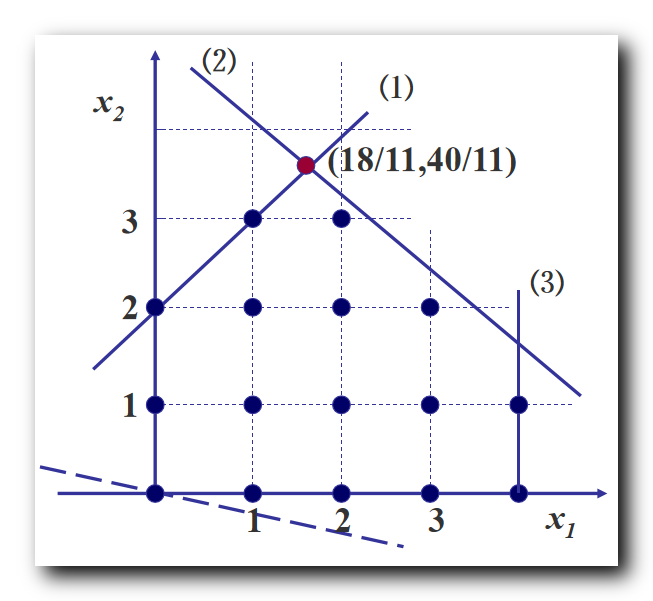使用图示法解得上述 松弛问题 最优解 $\begin{cases} \rm x_1 = \cfrac{18}{11} \approx 1.64 \\\\ \rm x_2 = \cfrac{40}{11} \approx 3.64 \end{cases}$ , 将上述最优解代入约束方程 ,
得到整数规划最小值 $\rm min W = -x_1 -5 x_2 = - \cfrac{218}{11} \approx -19.8$

如果上述 松弛问题 最优解 是整数 , 则该整数线性规划的最优解就是 松弛问题 的最优解 ;
上述 松弛问题 $\rm LP$ 最优解不是整数 , 这里需要进行 分支 操作 , 分成两个 分支松弛问题 $\rm LP1$ 和 $\rm LP2$ ;

3、第一次分支操作

分支操作 : 任选一个 非整数解变量 $x_i$ , 在 松弛问题 中加上约束 , $x_i \leq [x_i]$ 和 $x_i \geq [x_i] + 1$  , 形成 两个新的 松弛问题 , 就是两个分支 ;

选择 非整数取值的变量 $x_1 = \cfrac{18}{11} \approx 1.64$ , 作为分支变量 ,
$x_i \leq [x_i]$ 对应的 分支新增约束条件是 $x_1 \leq 1$ ;
$x_i \geq [x_i] + 1$ 对应的 分支新增约束条件是 $x_1 \geq 2$ ;

在 $\rm LP1$ 分支松弛问题中加入 $x_1 \leq 1$ 条件后为 :
$\begin{array}{lcl} \rm min W = -x_1 -5 x_2 \\\\ \rm s.t\begin{cases} \rm x_1 - x_2 \geq -2 \\\\ \rm 5x_1 + 6x_2 \leq 30 \\\\ \rm x_1 \leq 4 \\\\ \rm x_1 \leq 1 \\\\ \rm x_1, x_2 \geq 0 \end{cases}\end{array}$
求上述 $\rm LP1$ 分支的最优解  $\begin{cases} \rm x_1 = 1 \\\\ \rm x_2 = 3 \end{cases}$ , 将上述最优解代入约束方程 ,
得到整数规划最小值 $\rm min W = -x_1 -5 x_2 = - 16$
$\rm LP1$ 分支的界就是 $-16$ ;

在 $\rm LP2$ 分支松弛问题中加入 $x_1 \geq 2$ 条件后为 :
$\begin{array}{lcl} \rm min W = -x_1 -5 x_2 \\\\ \rm s.t\begin{cases} \rm x_1 - x_2 \geq -2 \\\\ \rm 5x_1 + 6x_2 \leq 30 \\\\ \rm x_1 \leq 4 \\\\ \rm x_1 \geq 2 \\\\ \rm x_1, x_2 \geq 0 \end{cases}\end{array}$
求上述 $\rm LP2$ 分支的最优解  $\begin{cases} \rm x_1 = 2 \\\\ \rm x_2 = \cfrac{10}{3} \end{cases}$ , 将上述最优解代入约束方程 ,
得到整数规划最小值 $\rm min W = -x_1 -5 x_2 = - \cfrac{56}{3} \approx -18.7$
$\rm LP2$ 分支的目标值是 $-18.7$ ;

$\rm LP1$ 分支的最优解时整数 , 界 是 $-16$ ,
$\rm LP2$ 分支目标值还不是整数 , 因此需要继续分支 ;

判定某个分支 松弛问题 是否继续向下分支的依据 :
① 根据整最优解是否是整数判定 : 首先看 分支 松弛问题 最优解 是否是整数 , 如果是那就停下来 , 如果不是继续向下分支 ;
② 根据界的优劣判定 ( 定界思想 ) : 是否继续向下分支 , 还需要看 界 的值 , 通过该 界 的值 , 讨论是否继续向下分支 ;

分支条件 : 如果 本分支的界 比 另外一个分支的界  要好 , 则继续分支下去 ;
不分支条件 : 如果本分支的界比另外一个分支的界差 , 那么本分支就不再向下分支了 ;

4、第二次分支操作

$\rm LP2$ 继续向下分支 , $x_1$ 变量已经是整数变量了 ,
这里 选择 非整数取值的变量 $x_2 = \cfrac{10}{3} \approx 3.33$ , 作为分支变量 ,
$x_i \leq [x_i]$ 对应的 分支新增约束条件是 $x_2 \leq 3$ ;
$x_i \geq [x_i] + 1$ 对应的 分支新增约束条件是 $x_2 \geq 4$ ;

这里得到了 $\rm LP2$ 分支下的两个 分支松弛问题 $\rm LP21$ 和 $\rm LP22$ ;
在 $\rm LP21$ 分支松弛问题中加入 $x_2 \leq 3$ 条件后为 :
$\begin{array}{lcl} \rm min W = -x_1 -5 x_2 \\\\ \rm s.t\begin{cases} \rm x_1 - x_2 \geq -2 \\\\ \rm 5x_1 + 6x_2 \leq 30 \\\\ \rm x_1 \leq 4 \\\\ \rm x_1 \geq 2 \\\\ \rm x_2 \leq 3 \\\\ \rm x_1, x_2 \geq 0 \end{cases}\end{array}$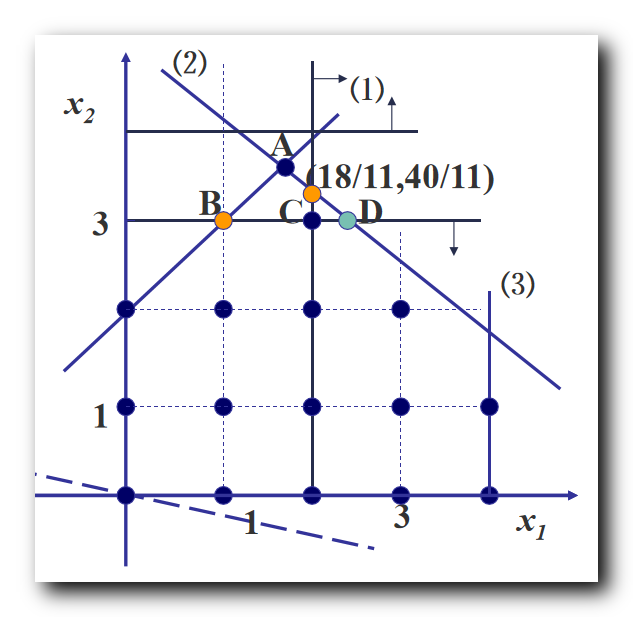求上述 $\rm LP21$ 分支的最优解  $\begin{cases} \rm x_1 = \cfrac{12}{5} = 2.4 \\\\ \rm x_2 = 3 \end{cases}$ , 将上述最优解代入约束方程 ,
得到整数规划最小值 $\rm min W = -x_1 -5 x_2 = - 17.4$
$\rm LP21$ 分支的最优解不是整数 , 而且比 $\rm LP1$ 分支的界 $-16$ 要好 , 可需要继续分支 ;

在 $\rm LP22$ 分支松弛问题中加入 $x_2 \geq 4$ 条件后为 :
$\begin{array}{lcl} \rm min W = -x_1 -5 x_2 \\\\ \rm s.t\begin{cases} \rm x_1 - x_2 \geq -2 \\\\ \rm 5x_1 + 6x_2 \leq 30 \\\\ \rm x_1 \leq 4 \\\\ \rm x_1 \geq 2 \\\\ \rm x_2 \geq 4 \\\\ \rm x_1, x_2 \geq 0 \end{cases}\end{array}$
上述 $\rm LP22$ 分支 没有可行解 , 直接停止 ;

5、第三次分支操作

$\rm LP21$ 继续向下分支 ,
这里 选择 非整数取值的变量 $x_1 = \cfrac{12}{5} = 2.4$ , 作为分支变量 ,
$x_i \leq [x_i]$ 对应的 分支新增约束条件是 $x_1 \leq 2$ ;
$x_i \geq [x_i] + 1$ 对应的 分支新增约束条件是 $x_1 \geq 3$ ;
这里得到了 $\rm LP21$ 分支下的两个 分支松弛问题 $\rm LP211$ 和 $\rm LP212$ ;

在 $\rm LP211$ 分支松弛问题中加入 $x_1 \leq 2$ 条件后为 :
$\begin{array}{lcl} \rm min W = -x_1 -5 x_2 \\\\ \rm s.t\begin{cases} \rm x_1 - x_2 \geq -2 \\\\ \rm 5x_1 + 6x_2 \leq 30 \\\\ \rm x_1 \leq 4 \\\\ \rm x_1 \geq 2 \\\\ \rm x_1 \leq 2 \\\\ \rm x_2 \leq 3 \\\\ \rm x_1, x_2 \geq 0 \end{cases}\end{array}$
求上述 $\rm LP211$ 分支的最优解  $\begin{cases} \rm x_1 = 2 \\\\ \rm x_2 = 3 \end{cases}$ , 将上述最优解代入约束方程 ,
得到整数规划最小值 $\rm min W = -x_1 -5 x_2 = - 17$
$\rm LP21$ 分支的最优解是整数 , 而且比 $\rm LP1$ 分支的界 $-16$ 要好 , 是当前最好的 界 ;
因此这里将 界 更新为 $-17$ ;

在 $\rm LP212$ 分支松弛问题中加入 $x_1 \geq 3$ 条件后为 :
$\begin{array}{lcl} \rm min W = -x_1 -5 x_2 \\\\ \rm s.t\begin{cases} \rm x_1 - x_2 \geq -2 \\\\ \rm 5x_1 + 6x_2 \leq 30 \\\\ \rm x_1 \leq 4 \\\\ \rm x_1 \geq 2 \\\\ \rm x_1 \geq 3 \\\\ \rm x_2 \leq 3 \\\\ \rm x_1, x_2 \geq 0 \end{cases}\end{array}$
求上述 $\rm LP212$ 分支的最优解  $\begin{cases} \rm x_1 =3 \\\\ \rm x_2 = 2.5 \end{cases}$ , 将上述最优解代入约束方程 ,
得到整数规划最小值 $\rm min W = -x_1 -5 x_2 = - 15.5$
定界 : $\rm LP212$ 分支的最优解不是整数 , 其目标值 $- 15.5$ 要比当前的界 $-17$ 要差 , 因此该分支直接裁减掉 ;

6、整数规划最优解

该整数规划 $\rm IP$ 的最优解  $\begin{cases} \rm x_1 = 2 \\\\ \rm x_2 = 3 \end{cases}$ , 将上述最优解代入约束方程 ,
得到整数规划最小值 $\rm min W = -x_1 -5 x_2 = - 17$

分支记录如下 :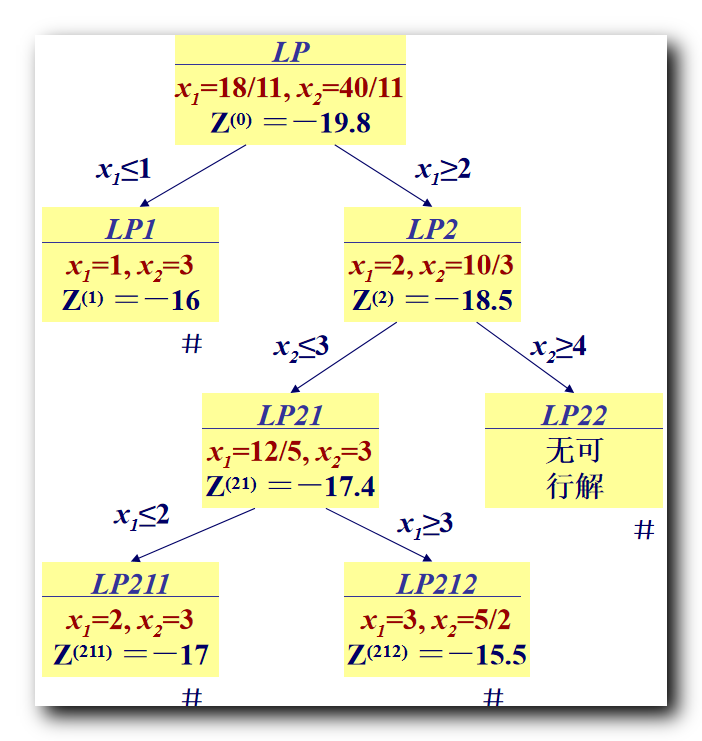展开全文整数规划
• 分支定界分支方法 分支定界 (Branch and bound) Branch and bound is a state space search method that can be termed as an improved form of backtracking. 分支定界是一种状态空间搜索方法，可以称为回溯的一种...
分支定界分支方法 分支定界 (Branch and bound)
Branch and bound is a state space search method that can be termed as an improved form of backtracking. 分支定界是一种状态空间搜索方法，可以称为回溯的一种改进形式。 It is suitable for solving the combinatorial optimization problem. 适用于解决组合优化问题。 These problems have an enumerable finite feasible solution. 这些问题具有有限的可行解。
In this approach, a set of feasible solutions are partitioned and the subsets that do not have an optimal solution are deleted for further consideration. Thus, the branch and bound has two major steps:
在这种方法中，对一组可行解进行了划分，并删除了没有最优解的子集，以供进一步考虑。 因此，分支和绑定有两个主要步骤：
Branching
Bounding
The following are the steps that are repeated to solve any problem are:
以下是解决任何问题重复执行的步骤：
1)分支 (1) Branching)
Branching is the first step of the branch and bound technique. This technique involves division of the main problem into two or more subproblems. These subproblems are exclusive and independent of each other. In addition, they are similar to the original problem but smaller in size i.e. the operation applied to the main problem can also be applied to these subproblems.
分支是分支定界技术的第一步。 该技术涉及将主要问题分为两个或多个子问题。 这些子问题是排他的，彼此独立。 此外，它们与原始问题相似，但尺寸较小，即，应用于主要问题的操作也可以应用于这些子问题。
2)边界 (2) Bounding)
Bounding operation restricts the state space tree to grow exponentially.
边界操作限制状态空间树成倍增长。
Branch and bound is the extension of backtracking which is used to solve combinatorial optimize problem. This is more useful because in this the branches are more optimally find as it uses the breath first search so, that our search is more optimal and state space tree is more compact. This can be solved by using the following:
分支定界是回溯的扩展，用于解决组合优化问题。 这更有用，因为在这种情况下，由于它使用呼吸优先搜索，因此可以更好地找到分支，从而使我们的搜索更加优化，状态空间树也更加紧凑。 可以使用以下方法解决：
FIFO - (First In First Out): The list of live nodes is a first - in - first - out the list. Example - queue. FIFO-(先进先出)：活动节点列表是先进先出列表。 示例队列 。 LIFO - (Last In Last Out): The list of the live nodes is a last - in - first - out the list. Example - stack. LIFO-(后进后出)：活动节点的列表是后进先出列表。 示例堆栈 。 LCS - (Least Cost Search): In this, we give the preference to an answer node with minimum cost. LCS-(最低成本搜索)：在这种情况下，我们将优先考虑的是成本最低的答案节点。 The branch and bound is that state space method in which all the children’s of the E-node are generated before any other live node can become the E-node. The two graph strategies i.e. the Breath first search and depth-first search, in which the exploration of a new node cannot begin until the node currently being explored is fully explored. Bounding function is used to avoid the generation of subtrees that do not contain an answer node. Branch and bound check the state space tree complexity for an optimal value and potential solutions are always obtained. This branch and bound is mostly used as it makes more compact state space tree.

分支定界是一种状态空间方法，其中在任何其他活动节点都可以成为E节点之前生成E节点的所有子节点。 两种图策略，即“呼吸优先”搜索和“深度优先”搜索，其中直到充分探索当前正在探索的节点之前，才可以开始探索新节点。 边界函数用于避免生成不包含答案节点的子树。 分支定界检查状态空间树的复杂性以获得最佳值，并始终获得潜在的解决方案。 这个分支和边界最常用，因为它使状态空间树更紧凑。
翻译自: https://www.includehelp.com/algorithms/branch-and-bound.aspx分支定界分支方法
展开全文python 算法 java 数据结构 机器学习
• 分支定界法（branch and bound）是一种求解整数规划问题的最常用算法。这种方法不但可以求解纯整数规划，还可以求解混合整数规划问题。分支定界法是一种搜索与迭代的方法，选择不同的分支变量和子问题进行分支。数学建模
• 此演示展示了最近邻匈牙利方法（munkres 算法）的单个步骤，用于分配问题、对称或非对称成本矩阵的分支定界。 显示了分支定界算法的树，用户可以选择更多或更少的细节。 文件提供了4个例子，也可以输入自己的例子。...matlab
• 一、分支定界法求整数规划示例、 二、求整数规划的松弛问题及最优解、 三、第一次分支操作、 四、第二次分支操作、 五、第三次分支操作、 六、整数规划最优解
文章目录一、分支定界法求整数规划示例二、求整数规划的松弛问题及最优解三、第一次分支操作四、第二次分支操作五、第三次分支操作六、整数规划最优解

一、分支定界法求整数规划示例

使用 分支定界法 求如下整数规划 :
$\begin{array}{lcl} \rm min W = -x_1 -5 x_2 \\\\ \rm s.t\begin{cases} \rm x_1 - x_2 \geq -2 \\\\ \rm 5x_1 + 6x_2 \leq 30 \\\\ \rm x_1 \leq 4 \\\\ \rm x_1, x_2 \geq 0 \ 并且为整数 \end{cases}\end{array}$

二、求整数规划的松弛问题及最优解

求上述整数规划 ( $\rm IP$ ) 的松弛问题 ( $\rm LP$ ) : 去掉整数限制即可得到一个一般的线性规划 ;
$\begin{array}{lcl} \rm min W = -x_1 -5 x_2 \\\\ \rm s.t\begin{cases} \rm x_1 - x_2 \geq -2 \\\\ \rm 5x_1 + 6x_2 \leq 30 \\\\ \rm x_1 \leq 4 \\\\ \rm x_1, x_2 \geq 0 \end{cases}\end{array}$
可以使用单纯形法求上述线性规划的最优解 , 本次使用图示法 , 求的最优化 ;使用图示法解得上述 松弛问题 最优解 $\begin{cases} \rm x_1 = \cfrac{18}{11} \approx 1.64 \\\\ \rm x_2 = \cfrac{40}{11} \approx 3.64 \end{cases}$ , 将上述最优解代入约束方程 ,
得到整数规划最小值 $\rm min W = -x_1 -5 x_2 = - \cfrac{218}{11} \approx -19.8$

如果上述 松弛问题 最优解 是整数 , 则该整数线性规划的最优解就是 松弛问题 的最优解 ;
上述 松弛问题 $\rm LP$ 最优解不是整数 , 这里需要进行 分支 操作 , 分成两个 分支松弛问题 $\rm LP1$ 和 $\rm LP2$ ;

三、第一次分支操作

分支操作 : 任选一个 非整数解变量 $x_i$ , 在 松弛问题 中加上约束 , $x_i \leq [x_i]$ 和 $x_i \geq [x_i] + 1$  , 形成 两个新的 松弛问题 , 就是两个分支 ;

选择 非整数取值的变量 $x_1 = \cfrac{18}{11} \approx 1.64$ , 作为分支变量 ,
$x_i \leq [x_i]$ 对应的 分支新增约束条件是 $x_1 \leq 1$ ;
$x_i \geq [x_i] + 1$ 对应的 分支新增约束条件是 $x_1 \geq 2$ ;

在 $\rm LP1$ 分支松弛问题中加入 $x_1 \leq 1$ 条件后为 :
$\begin{array}{lcl} \rm min W = -x_1 -5 x_2 \\\\ \rm s.t\begin{cases} \rm x_1 - x_2 \geq -2 \\\\ \rm 5x_1 + 6x_2 \leq 30 \\\\ \rm x_1 \leq 4 \\\\ \rm x_1 \leq 1 \\\\ \rm x_1, x_2 \geq 0 \end{cases}\end{array}$
求上述 $\rm LP1$ 分支的最优解  $\begin{cases} \rm x_1 = 1 \\\\ \rm x_2 = 3 \end{cases}$ , 将上述最优解代入约束方程 ,
得到整数规划最小值 $\rm min W = -x_1 -5 x_2 = - 16$
$\rm LP1$ 分支的界就是 $-16$ ;

在 $\rm LP2$ 分支松弛问题中加入 $x_1 \geq 2$ 条件后为 :
$\begin{array}{lcl} \rm min W = -x_1 -5 x_2 \\\\ \rm s.t\begin{cases} \rm x_1 - x_2 \geq -2 \\\\ \rm 5x_1 + 6x_2 \leq 30 \\\\ \rm x_1 \leq 4 \\\\ \rm x_1 \geq 2 \\\\ \rm x_1, x_2 \geq 0 \end{cases}\end{array}$
求上述 $\rm LP2$ 分支的最优解  $\begin{cases} \rm x_1 = 2 \\\\ \rm x_2 = \cfrac{10}{3} \end{cases}$ , 将上述最优解代入约束方程 ,
得到整数规划最小值 $\rm min W = -x_1 -5 x_2 = - \cfrac{56}{3} \approx -18.7$
$\rm LP2$ 分支的目标值是 $-18.7$ ;

$\rm LP1$ 分支的最优解时整数 , 界 是 $-16$ ,
$\rm LP2$ 分支目标值还不是整数 , 因此需要继续分支 ;

判定某个分支 松弛问题 是否继续向下分支的依据 :
① 根据整最优解是否是整数判定 : 首先看 分支 松弛问题 最优解 是否是整数 , 如果是那就停下来 , 如果不是继续向下分支 ;
② 根据界的优劣判定 ( 定界思想 ) : 是否继续向下分支 , 还需要看 界 的值 , 通过该 界 的值 , 讨论是否继续向下分支 ;

分支条件 : 如果 本分支的界 比 另外一个分支的界  要好 , 则继续分支下去 ;
不分支条件 : 如果本分支的界比另外一个分支的界差 , 那么本分支就不再向下分支了 ;

四、第二次分支操作

$\rm LP2$ 继续向下分支 , $x_1$ 变量已经是整数变量了 ,
这里 选择 非整数取值的变量 $x_2 = \cfrac{10}{3} \approx 3.33$ , 作为分支变量 ,
$x_i \leq [x_i]$ 对应的 分支新增约束条件是 $x_2 \leq 3$ ;
$x_i \geq [x_i] + 1$ 对应的 分支新增约束条件是 $x_2 \geq 4$ ;

这里得到了 $\rm LP2$ 分支下的两个 分支松弛问题 $\rm LP21$ 和 $\rm LP22$ ;
在 $\rm LP21$ 分支松弛问题中加入 $x_2 \leq 3$ 条件后为 :
$\begin{array}{lcl} \rm min W = -x_1 -5 x_2 \\\\ \rm s.t\begin{cases} \rm x_1 - x_2 \geq -2 \\\\ \rm 5x_1 + 6x_2 \leq 30 \\\\ \rm x_1 \leq 4 \\\\ \rm x_1 \geq 2 \\\\ \rm x_2 \leq 3 \\\\ \rm x_1, x_2 \geq 0 \end{cases}\end{array}$求上述 $\rm LP21$ 分支的最优解  $\begin{cases} \rm x_1 = \cfrac{12}{5} = 2.4 \\\\ \rm x_2 = 3 \end{cases}$ , 将上述最优解代入约束方程 ,
得到整数规划最小值 $\rm min W = -x_1 -5 x_2 = - 17.4$
$\rm LP21$ 分支的最优解不是整数 , 而且比 $\rm LP1$ 分支的界 $-16$ 要好 , 可需要继续分支 ;

在 $\rm LP22$ 分支松弛问题中加入 $x_2 \geq 4$ 条件后为 :
$\begin{array}{lcl} \rm min W = -x_1 -5 x_2 \\\\ \rm s.t\begin{cases} \rm x_1 - x_2 \geq -2 \\\\ \rm 5x_1 + 6x_2 \leq 30 \\\\ \rm x_1 \leq 4 \\\\ \rm x_1 \geq 2 \\\\ \rm x_2 \geq 4 \\\\ \rm x_1, x_2 \geq 0 \end{cases}\end{array}$
上述 $\rm LP22$ 分支 没有可行解 , 直接停止 ;

五、第三次分支操作

$\rm LP21$ 继续向下分支 ,
这里 选择 非整数取值的变量 $x_1 = \cfrac{12}{5} = 2.4$ , 作为分支变量 ,
$x_i \leq [x_i]$ 对应的 分支新增约束条件是 $x_1 \leq 2$ ;
$x_i \geq [x_i] + 1$ 对应的 分支新增约束条件是 $x_1 \geq 3$ ;
这里得到了 $\rm LP21$ 分支下的两个 分支松弛问题 $\rm LP211$ 和 $\rm LP212$ ;

在 $\rm LP211$ 分支松弛问题中加入 $x_1 \leq 2$ 条件后为 :
$\begin{array}{lcl} \rm min W = -x_1 -5 x_2 \\\\ \rm s.t\begin{cases} \rm x_1 - x_2 \geq -2 \\\\ \rm 5x_1 + 6x_2 \leq 30 \\\\ \rm x_1 \leq 4 \\\\ \rm x_1 \geq 2 \\\\ \rm x_1 \leq 2 \\\\ \rm x_2 \leq 3 \\\\ \rm x_1, x_2 \geq 0 \end{cases}\end{array}$
求上述 $\rm LP211$ 分支的最优解  $\begin{cases} \rm x_1 = 2 \\\\ \rm x_2 = 3 \end{cases}$ , 将上述最优解代入约束方程 ,
得到整数规划最小值 $\rm min W = -x_1 -5 x_2 = - 17$
$\rm LP21$ 分支的最优解是整数 , 而且比 $\rm LP1$ 分支的界 $-16$ 要好 , 是当前最好的 界 ;
因此这里将 界 更新为 $-17$ ;

在 $\rm LP212$ 分支松弛问题中加入 $x_1 \geq 3$ 条件后为 :
$\begin{array}{lcl} \rm min W = -x_1 -5 x_2 \\\\ \rm s.t\begin{cases} \rm x_1 - x_2 \geq -2 \\\\ \rm 5x_1 + 6x_2 \leq 30 \\\\ \rm x_1 \leq 4 \\\\ \rm x_1 \geq 2 \\\\ \rm x_1 \geq 3 \\\\ \rm x_2 \leq 3 \\\\ \rm x_1, x_2 \geq 0 \end{cases}\end{array}$
求上述 $\rm LP212$ 分支的最优解  $\begin{cases} \rm x_1 =3 \\\\ \rm x_2 = 2.5 \end{cases}$ , 将上述最优解代入约束方程 ,
得到整数规划最小值 $\rm min W = -x_1 -5 x_2 = - 15.5$
定界 : $\rm LP212$ 分支的最优解不是整数 , 其目标值 $- 15.5$ 要比当前的界 $-17$ 要差 , 因此该分支直接裁减掉 ;

六、整数规划最优解

该整数规划 $\rm IP$ 的最优解  $\begin{cases} \rm x_1 = 2 \\\\ \rm x_2 = 3 \end{cases}$ , 将上述最优解代入约束方程 ,
得到整数规划最小值 $\rm min W = -x_1 -5 x_2 = - 17$

分支记录如下 :展开全文整数规划
• ## 分支定界算法

万次阅读 多人点赞 2019-09-05 08:45:29
分支定界算法(Branch and bound，简称为 BB、B&B, or BnB)始终围绕着一颗搜索树进行的，我们将原问题看作搜索树的根节点，从这里出发，分支的含义就是将大的问题分割成小的问题。大问题可以看成是搜索树的父节点...
•研究论文
• ## 分支定界法(matlab实现)

万次阅读 多人点赞 2019-06-26 19:28:23
分支定界法 背景 今天利用matlab来实现求解完全整数规划问题和混合整数规划问题的分支定界法。 基本理论 分支定界法：用以求解整数规划问题的一种方法。 求解步骤： 求出该整数规划问题对应的原线性规划问题的最优...matlab
• 分支定界法python实现，一个例子，可以供研究学习用
•研究论文
• 分支定界法（branch and bound）是一种求解离散数据组合的最优化问题。该算法执行的效率取决于你所找的问题解空间的上下界，如果找到一个很紧凑的上下界进行剪枝操作，该算法的执行效率会非常高，因此它是最有可能在...
•研究论文
• 最小二乘回归的子集（特征）选择是一个常见问题，它是组合问题，因此在计算上是 NP ... 此代码提供了一种使用改进的向下分支定界方法来有效解决此问题的工具。 该算法的应用之一是选择全局最优控制变量进行自优化控制。matlab
• 分支定界法.分支定界法.分支定界法.分支定界法.分支定界法.分支定界法.分支定界法.分支定界法.分支定界法.分支定界法.分支定界法.分支定界法.
• 分支定界算法与TSP 1）分支定界算法： 估价函数：用于确定决策分支的取值界限 分支：每一步决策有多种决策方案，每一个方案都构成一个决策分支。 定界： 在当前决策情况下，估计当前分支完成全部决策时的最优取值 ...算法 TSP问题
• cartographer 代码思想解读（2）- 分支定界快速相关匹配： https://blog.csdn.net/jiajiading/article/details/108398423?utm_medium=distribute.pc_relevant.none-task-blog-OPENSEARCH-3.control&dist_request...
• 整数规划之分支定界法 Reference: 运筹学之分支定界法 整数规划问题和一般的线性规划问题很类似，唯一的不同点在于可行解必须是整数。 这是因为对于某些实际问题，必须要求全部解或者至少部分解为整数，如，所求的...
•研究论文
• 分支定界法的基本思想是对有约束条件的最优化问题的所有可行解（数目有限）空间进行搜索 2. 什么是分支定界分支定界算法始终围绕着一颗搜索树进行的，我们将原始问题看出搜索树的根节点，从这里出发，分支的含义...
• B3MSV 双向分支定界 (B3) 子集选择使用最小奇异值 (MSV) 作为标准。 考虑以下子集选择问题： 给定一个高 (mxn, m>n) 矩阵 A，找到 A 的 n 行，使得生成的 nxn 方形子矩阵在所有可能的 nxn 子矩阵中具有最大的 MSV...